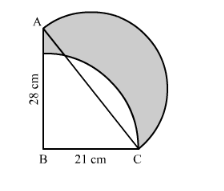# In the following figure, ABC is a right-angled triangle,Question:

In the following figure, ABC is a right-angled triangle, ∠B = 90°, AB = 28 cm and BC = 21 cm. With AC as diameter a semicircle is drawn and with BC as radius a quarter circle is drawn. Find the area of the shaded region correct to two decimal places.Solution:

We have given two semi-circles and one circle.

Area of the shaded region = area of semicircle with diameter AC + area of right angled triangle ABC − area of sector

First we will find the hypotenuse of right angled triangle ABC.

$A C^{2}=A B^{2}+B C^{2}$

$\therefore A C^{2}=28^{2}+21^{2}$

$\therefore A C^{2}=784+441$

$\therefore A C^{2}=1225$

$\therefore A C=35$

$\therefore$ Area of the shaded region $=\frac{\pi \times 17.5 \times 17.5}{2}+\frac{1}{2} \times 28 \times 21-\frac{\theta}{360} \times \pi \times 21 \times 21$

$\therefore$ Area of the shaded region $=\frac{\pi \times 17.5 \times 17.5}{2}+14 \times 21-\frac{1}{4} \times \pi \times 21 \times 21$

Substituting $\pi=\frac{22}{7}$ we get,

$\therefore$ Area of the shaded region $=\frac{\frac{22}{7} \times 17.5 \times 17.5}{2}+14 \times 21-\frac{1}{4} \times \frac{22}{7} \times 21 \times 21$

$\therefore$ Area of the shaded region $=\frac{962.5}{2}+14 \times 21-\frac{1}{2} \times 11 \times 3 \times 21$

$\therefore$ Area of the shaded region $=481.25+294-346.5$

$\therefore$ Area of the shaded region $=428.75$

Therefore, area of shaded region is $428.75 \mathrm{~cm}^{2}$.